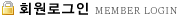회원로그인 아이디 비밀번호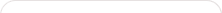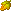아리랑가요(무료음악채널)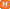뽕짝아가씨(무료음악채널)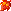okTrot(무료음악채널)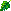전통가요(무료음악채널)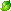미스트롯(무료음악채널)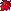온라이브스토어(앱다운로드)환상의뽕짝파티 무료음악명작트로트메들리 무료음악트로트메들리관광 무료음악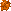동영상노래방가사검색POP Player신나는 고스톱컴퓨터 오목작은 음악다방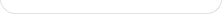number format 함수 사용법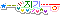10년 전
 number_format 함수를 사용하세요.. 설명서와 사용예입니다. Purpose: Format a number with grouped thousands Usage: string number_format (float number [, int decimals [, string dec_point [, string thousands_sep]]]) Availability: PHP 3, PHP 4 >= 4.0.0 number_format() returns a formatted version of number. This function accepts either one, two or four parameters (not three): If only one parameter is given, Number will be formatted without decimals, but with a comma (",") between every group of thousands. If two parameters are given, number will be formatted with decimals decimals with a dot (".") in front, and a comma (",") between every group of thousands. If all four parameters are given, number will be formatted with decimals decimals, dec_point instead of a dot (".") before the decimals and thousands_sep instead of a comma (",") between every group of thousands. Note: Only the first character of thousands_sep is used. For example, if you use foo as thousands_sep on the number 1000, number_format() will return 1f000. Example 1. number_format() Example For instance, French notation usually use two decimals, comma (',') as decimal separator, and space (' ') as thousand separator. This is achieved with this line : \$number = 1234.56; // english notation (default) \$english_format_number = number_format(\$number); // 1,234.56 // French notation \$nombre_format_francais = number_format(\$number, 2, ',', ' '); // 1 234,56 \$number = 1234.5678; // english notation without thousands seperator \$english_format_number = number_format(\$number, 2, '.', ''); // 1234.56 ?>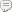추천 : 185
 번호 제목 3,020[HTML5]뮤직트로트 부산광역시부산진구 가야동 ㅣ 개인정보취급방침 Copyright (C) musictrot All rights reserved.